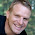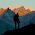How To Create Speed Testing Script Using Python Programming Language

Hello Friends,

Today's   I Will Show You How To Create Python Script for Measuring Our Average Internet Speed.  Ho,  I Know You Are Thinking That This Is not important Topic. But Believe me many time, we really needs this type of script for testing our internet connection speed.  so, don't worry and keep reading because today you will see how we can measure  our downloading speed.                       but first, if you are new visitor then first check our index.

So, Let's Start.

Here, I am Sharing My Demo Codes But If You Want More Better Example Then, You Can Modify these codes yourself or Download This Script From My GitHub repository (link given at the end of these codes ).

 ``` 1 2 3 4 5 6 7 8 9 10 11 12 13 14 15 16 17 18 19 20 21 22 23 24 25 26 27 28 29 30 31 32 33 34 35 36 37 38 39 40 41 42 43 44 45 46``` ```#!/usr/bin/python # -*- coding: utf-8 -*- # ---------------- READ ME --------------------------------------------- # This Script is Created Only For Practise And Educational Purpose Only # This Script Is Created For https://bitforestinfo.blogspot.com # This Script is Written By __author__=''' ###################################################### By S.S.B Group ###################################################### Suraj Singh Admin S.S.B Group surajsinghbisht054@gmail.com https://bitforestinfo.blogspot.in/ Note: We Feel Proud To Be Indian ###################################################### ''' # Import Module import urllib2 import time import sys def main(multi_times): starting_check_point = time.time() # Timer Starting Point print "[+] Test Started On : ", time.ctime(starting_check_point) download_data = [] # For Collecting Downloaded Data for i in range(multi_times): # Use Any Website Page data = urllib2.urlopen("https://en.wikipedia.org/wiki/Internet").read() download_data.append(sys.getsizeof(data)) # Calculating WebPage Size ending_check_point = time.time() # Time Closing Point print "[+] Test Closed On : ",time.ctime(ending_check_point) total_data_size = sum(download_data) # Calculating Total Size of Downloaded data total_time_used = ending_check_point - starting_check_point # Calculating Total Time one_byte_equal_to = 1024 speed = int(total_data_size/total_time_used/one_byte_equal_to) # Calculating Average Speed print "[+] Average InterNet Speed : {} KB".format(speed) if __name__=='__main__': main(5) ```

Here, You Can See I am Showing Internet Speed Only In Kilo Bytes But You Want More ... Then Check This Example

 ``` 1 2 3 4 5 6 7 8 9 10``` ```import math def convert_size(size_bytes): if (size_bytes == 0): return '0B' size_name = ("B", "KB", "MB", "GB", "TB", "PB", "EB", "ZB", "YB") i = int(math.floor(math.log(size_bytes, 1024))) p = math.pow(1024, i) s = round(size_bytes/p, 2) return '%s %s' % (s, size_name[i]) ```

I Taken This Example From Here : http://stackoverflow.com/questions/5194057/better-way-to-convert-file-sizes-in-python

Warning I am Creating This Tutorial Only For Practise and Educational Purpose. I will not Take any type of responsibility about any illegal activities.

For Video Check This

And Subscribe Our Blog,

For Any Type of Suggestion Or Help
Contact me:
S.S.B
surajsinghbisht054@gmail.com

1.1.